# PID controller theory v2.1

Transfer function of the PID controller this library implements is: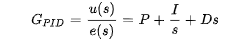Continuous PID is transformed to the discrete domain and can be described as a sum of three components: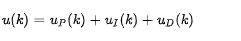proportional: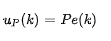integral: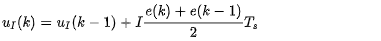derivative: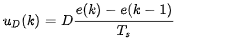Where the u(k) is the control signal (voltage Uq in our case) in moment k, e(k),e(k-1) is the tracking error in current moment k and previous step k-1. Tracking error presents the difference in between the target velocity value vd and measured velocity v.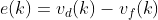## Implementation details

The PID algorithm is implemented in the SimpleFOClibrary in the PIDController class. The class is instantiated by specifying the parameters:

PIDController(float P, float I, float D, float ramp, float limit);


And the class has only one function:

// PID controller function
float PIDController::operator() (float error){
// calculate the time from the last call
unsigned long timestamp_now = _micros();
float Ts = (timestamp_now - timestamp_prev) * 1e-6;
// quick fix for strange cases (micros overflow)
if(Ts <= 0 || Ts > 0.5) Ts = 1e-3;

// u(s) = (P + I/s + Ds)e(s)
// Discrete implementations
// proportional part
// u_p  = P *e(k)
float proportional = P * error;
// Tustin transform of the integral part
// u_ik = u_ik_1  + I*Ts/2*(ek + ek_1)
float integral = integral_prev + I*Ts*0.5*(error + error_prev);
// antiwindup - limit the output voltage_q
integral = _constrain(integral, -limit, limit);
// Discrete derivation
// u_dk = D(ek - ek_1)/Ts
float derivative = D*(error - error_prev)/Ts;

// sum all the components
float output = proportional + integral + derivative;
// antiwindup - limit the output variable
output = _constrain(output, -limit, limit);

// limit the acceleration by ramping the output
float output_rate = (output - output_prev)/Ts;
if (output_rate > output_ramp)
output = output_prev + output_ramp*Ts;
else if (output_rate < -output_ramp)
output = output_prev - output_ramp*Ts;

// saving for the next pass
integral_prev = integral;
output_prev = output;
error_prev = error;
timestamp_prev = timestamp_now;
return output;
}


Therefore you can integrate the PID into your code very easily by just calling:

void setup(){
...
PIDController some_pid = PIDController{.....};
...
}
void loop(){
float control = some_pid(target-measurement);
}


This PID class is implemented in the BLDCMotor and StepperMotor class for handling the motion control velocity (motor.PID_velocity) and position (motor.P_angle). You can change the values parameters of these PID controllers by changing their public variables

// PID controller configuration structure
class PIDController
{
.....
float P; //!< Proportional gain
float I; //!< Integral gain
float D; //!< Derivative gain
....
};


For example:

motor.PID_velocity.P = 1;
motor.P_angle.P = 10;